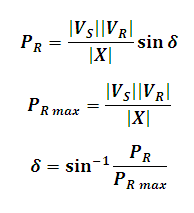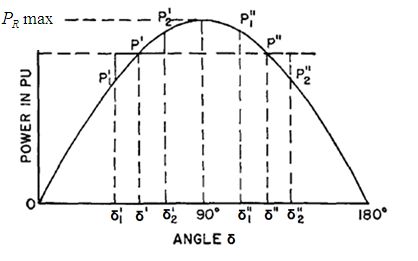# Power Transfer Angle Calculator

This Calctown Calculator calculates the power transfer angle of a transmission line.

ohm
kV
kV

#### Result

KWwhere

PR = Receiving end Power

PR max = Maximum Receiving end Power

X = Reactance of line

VS = Sending end Voltage

VR = Receiving end Voltage

δ = Power transfer angle